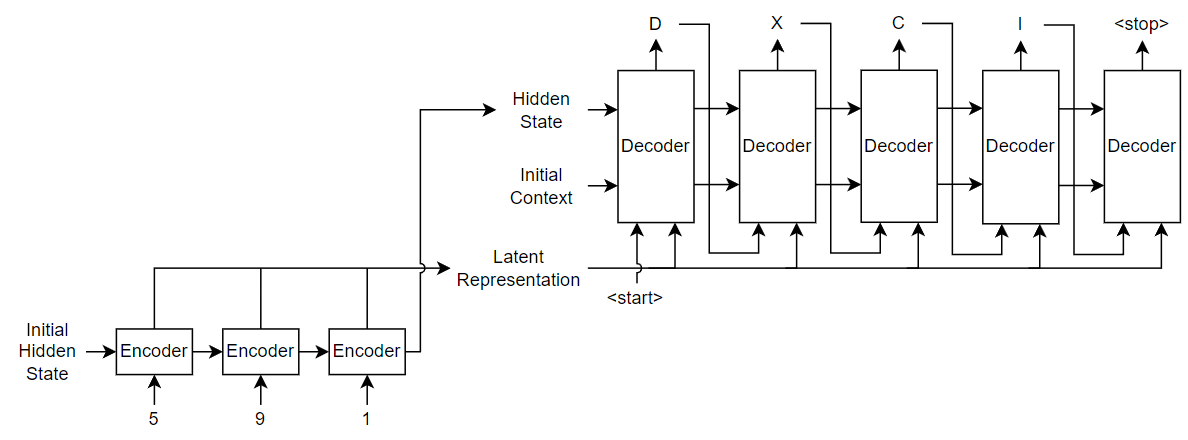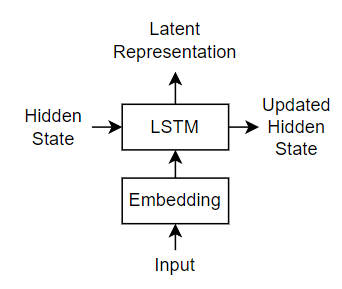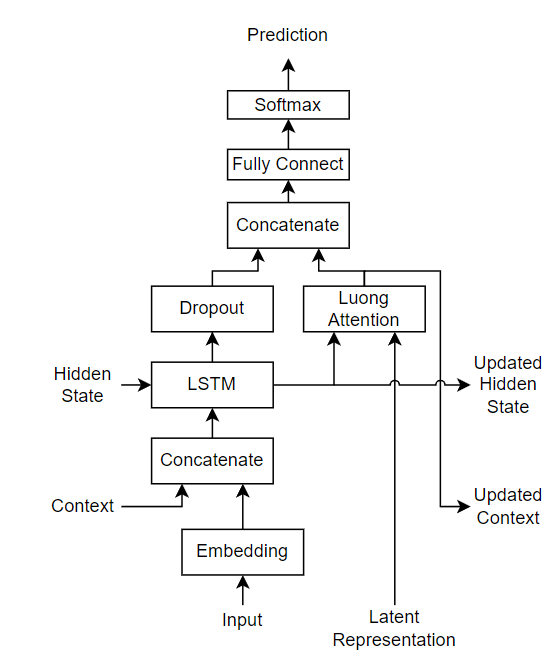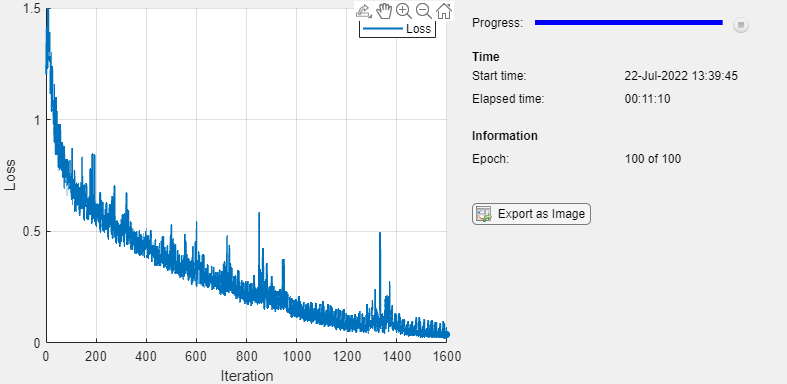# Sequence-to-Sequence Translation Using Attention

This example shows how to convert decimal strings to Roman numerals using a recurrent sequence-to-sequence encoder-decoder model with attention.

Recurrent encoder-decoder models have proven successful at tasks like abstractive text summarization and neural machine translation. The model consists of an encoder which typically processes input data with a recurrent layer such as LSTM, and a decoder which maps the encoded input into the desired output, typically with a second recurrent layer. Models that incorporate attention mechanisms into the models allows the decoder to focus on parts of the encoded input while generating the translation.For the encoder model, this example uses a simple network consisting of an embedding followed by two LSTM operations. Embedding is a method of converting categorical tokens into numeric vectors.For the decoder model, this example uses a network very similar to the encoder that contains two LSTMs. However, an important difference is that the decoder contains an attention mechanism. The attention mechanism allows the decoder to attend to specific parts of the encoder output.Download the decimal-Roman numeral pairs from `"romanNumerals.csv"`

```filename = fullfile("romanNumerals.csv"); options = detectImportOptions(filename, ... TextType="string", ... ReadVariableNames=false); options.VariableNames = ["Source" "Target"]; options.VariableTypes = ["string" "string"]; data = readtable(filename,options);```

Split the data into training and test partitions containing 50% of the data each.

```idx = randperm(size(data,1),500); dataTrain = data(idx,:); dataTest = data; dataTest(idx,:) = [];```

View some of the decimal-Roman numeral pairs.

`head(dataTrain)`
```ans=8×2 table Source Target ______ _________ "713" "DCCXIII" "752" "DCCLII" "434" "CDXXXIV" "641" "DCXLI" "68" "LXVIII" "87" "LXXXVII" "469" "CDLXIX" "715" "DCCXV" ```

### Preprocess Data

Preprocess the text data using the `transformText` function, listed at the end of the example. The `transformText` function preprocesses and tokenizes the input text for translation by splitting the text into characters and adding start and stop tokens. To translate text by splitting the text into words instead of characters, skip the first step.

```startToken = "<start>"; stopToken = "<stop>"; strSource = dataTrain.Source; documentsSource = transformText(strSource,startToken,stopToken);```

Create a `wordEncoding` object that maps tokens to a numeric index and vice-versa using a vocabulary.

`encSource = wordEncoding(documentsSource);`

Using the word encoding, convert the source text data to numeric sequences.

`sequencesSource = doc2sequence(encSource,documentsSource,PaddingDirection="none");`

Convert the target data to sequences using the same steps.

```strTarget = dataTrain.Target; documentsTarget = transformText(strTarget,startToken,stopToken); encTarget = wordEncoding(documentsTarget); sequencesTarget = doc2sequence(encTarget,documentsTarget,PaddingDirection="none");```

Sort the sequences by length. Training with the sequences sorted by increasing sequence length results in batches with sequences of approximately the same sequence length and ensures smaller sequence batches are used to update the model before longer sequence batches.

```sequenceLengths = cellfun(@(sequence) size(sequence,2),sequencesSource); [~,idx] = sort(sequenceLengths); sequencesSource = sequencesSource(idx); sequencesTarget = sequencesTarget(idx);```

Create `arrayDatastore` objects containing the source and target data and combine them using the `combine` function.

```sequencesSourceDs = arrayDatastore(sequencesSource,OutputType="same"); sequencesTargetDs = arrayDatastore(sequencesTarget,OutputType="same"); sequencesDs = combine(sequencesSourceDs,sequencesTargetDs);```

### Initialize Model Parameters

Initialize the model parameters. for both the encoder and decoder, specify an embedding dimension of 128, two LSTM layers with 200 hidden units, and dropout layers with random dropout with probability 0.05.

```embeddingDimension = 128; numHiddenUnits = 200; dropout = 0.05;```

#### Initialize Encoder Model Parameters

Initialize the weights of the encoding embedding using the Gaussian using the `initializeGaussian` function which is attached to this example as a supporting file. Specify a mean of 0 and a standard deviation of 0.01. To learn more, see Gaussian Initialization.

```inputSize = encSource.NumWords + 1; sz = [embeddingDimension inputSize]; mu = 0; sigma = 0.01; parameters.encoder.emb.Weights = initializeGaussian(sz,mu,sigma);```

Initialize the learnable parameters for the encoder LSTM operations:

• Initialize the input weights with the Glorot initializer using the `initializeGlorot` function which is attached to this example as a supporting file. To learn more, see Glorot Initialization.

• Initialize the recurrent weights with the orthogonal initializer using the `initializeOrthogonal` function which is attached to this example as a supporting file. To learn more, see Orthogonal Initialization.

• Initialize the bias with the unit forget gate initializer using the `initializeUnitForgetGate` function which is attached to this example as a supporting file. To learn more, see Unit Forget Gate Initialization.

Initialize the learnable parameters for the first encoder LSTM operation.

```sz = [4*numHiddenUnits embeddingDimension]; numOut = 4*numHiddenUnits; numIn = embeddingDimension; parameters.encoder.lstm1.InputWeights = initializeGlorot(sz,numOut,numIn); parameters.encoder.lstm1.RecurrentWeights = initializeOrthogonal([4*numHiddenUnits numHiddenUnits]); parameters.encoder.lstm1.Bias = initializeUnitForgetGate(numHiddenUnits);```

Initialize the learnable parameters for the second encoder LSTM operation.

```sz = [4*numHiddenUnits numHiddenUnits]; numOut = 4*numHiddenUnits; numIn = numHiddenUnits; parameters.encoder.lstm2.InputWeights = initializeGlorot(sz,numOut,numIn); parameters.encoder.lstm2.RecurrentWeights = initializeOrthogonal([4*numHiddenUnits numHiddenUnits]); parameters.encoder.lstm2.Bias = initializeUnitForgetGate(numHiddenUnits);```

#### Initialize Decoder Model Parameters

Initialize the weights of the encoding embedding using the Gaussian using the `initializeGaussian` function. Specify a mean of 0 and a standard deviation of 0.01.

```outputSize = encTarget.NumWords + 1; sz = [embeddingDimension outputSize]; mu = 0; sigma = 0.01; parameters.decoder.emb.Weights = initializeGaussian(sz,mu,sigma);```

Initialize the weights of the attention mechanism using the Glorot initializer using the `initializeGlorot` function.

```sz = [numHiddenUnits numHiddenUnits]; numOut = numHiddenUnits; numIn = numHiddenUnits; parameters.decoder.attn.Weights = initializeGlorot(sz,numOut,numIn);```

Initialize the learnable parameters for the decoder LSTM operations:

• Initialize the input weights with the Glorot initializer using the `initializeGlorot` function.

• Initialize the recurrent weights with the orthogonal initializer using the `initializeOrthogonal` function.

• Initialize the bias with the unit forget gate initializer using the `initializeUnitForgetGate` function.

Initialize the learnable parameters for the first decoder LSTM operation.

```sz = [4*numHiddenUnits embeddingDimension+numHiddenUnits]; numOut = 4*numHiddenUnits; numIn = embeddingDimension + numHiddenUnits; parameters.decoder.lstm1.InputWeights = initializeGlorot(sz,numOut,numIn); parameters.decoder.lstm1.RecurrentWeights = initializeOrthogonal([4*numHiddenUnits numHiddenUnits]); parameters.decoder.lstm1.Bias = initializeUnitForgetGate(numHiddenUnits);```

Initialize the learnable parameters for the second decoder LSTM operation.

```sz = [4*numHiddenUnits numHiddenUnits]; numOut = 4*numHiddenUnits; numIn = numHiddenUnits; parameters.decoder.lstm2.InputWeights = initializeGlorot(sz,numOut,numIn); parameters.decoder.lstm2.RecurrentWeights = initializeOrthogonal([4*numHiddenUnits numHiddenUnits]); parameters.decoder.lstm2.Bias = initializeUnitForgetGate(numHiddenUnits);```

Initialize the learnable parameters for the decoder fully connected operation:

• Initialize the weights with the Glorot initializer.

• Initialize the bias with zeros using the `initializeZeros` function which is attached to this example as a supporting file. To learn more, see Zeros Initialization.

```sz = [outputSize 2*numHiddenUnits]; numOut = outputSize; numIn = 2*numHiddenUnits; parameters.decoder.fc.Weights = initializeGlorot(sz,numOut,numIn); parameters.decoder.fc.Bias = initializeZeros([outputSize 1]);```

### Define Model Functions

Create the functions `modelEncoder` and `modelDecoder`, listed at the end of the example, that compute the outputs of the encoder and decoder models, respectively.

The `modelEncoder` function, listed in the Encoder Model Function section of the example, takes the input data, the model parameters, the optional mask that is used to determine the correct outputs for training and returns the model outputs and the LSTM hidden state.

The `modelDecoder` function, listed in the Decoder Model Function section of the example, takes the input data, the model parameters, the context vector, the LSTM initial hidden state, the outputs of the encoder, and the dropout probability and outputs the decoder output, the updated context vector, the updated LSTM state, and the attention scores.

### Define Model Loss Function

Create the function `modelLoss`, listed in the Model LossFunction section of the example, that takes the encoder and decoder model parameters, a mini-batch of input data and the padding masks corresponding to the input data, and the dropout probability and returns the loss and the gradients of the loss with respect to the learnable parameters in the models.

### Specify Training Options

Train with a mini-batch size of 32 for 75 epochs with a learning rate of 0.002.

```miniBatchSize = 32; numEpochs = 75; learnRate = 0.002;```

```gradientDecayFactor = 0.9; squaredGradientDecayFactor = 0.999;```

### Train Model

Train the model using a custom training loop. Use `minibatchqueue` to process and manage mini-batches of images during training. For each mini-batch:

• Use the custom mini-batch preprocessing function `preprocessMiniBatch` (defined at the end of this example) to find the lengths of all sequence in the mini-batch and pad the sequences to the same length as the longest sequence, for the source and target sequences, respectively.

• Permute the second and third dimensions of the padded sequences.

• Return the mini-batch variables unformatted `dlarray` objects with underlying data type `single`. All other outputs are arrays of data type `single`.

• Train on a GPU if one is available. Return all mini-batch variables on the GPU if one is available. Using a GPU requires Parallel Computing Toolbox™ and a supported GPU device. For information on supported devices, see GPU Support by Release.

The `minibatchqueue` object returns four output arguments for each mini-batch: the source sequences, the target sequences, the lengths of all source sequences in the mini-batch, and the sequence mask of the target sequences.

```numMiniBatchOutputs = 4; mbq = minibatchqueue(sequencesDs,numMiniBatchOutputs,... MiniBatchSize=miniBatchSize,... MiniBatchFcn=@(x,t) preprocessMiniBatch(x,t,inputSize,outputSize));```

Initialize the training progress plot.

```figure C = colororder; lineLossTrain = animatedline(Color=C(2,:)); ylim([0 inf]) xlabel("Iteration") ylabel("Loss") grid on```

Initialize the values for the `adamupdate` function.

```trailingAvg = []; trailingAvgSq = [];```

Train the model. For each mini-batch:

• Update the encoder and decoder model parameters using the `adamupdate` function.

• Update the training progress plot.

```iteration = 0; start = tic; % Loop over epochs. for epoch = 1:numEpochs reset(mbq); % Loop over mini-batches. while hasdata(mbq) iteration = iteration + 1; [X,T,sequenceLengthsSource,maskSequenceTarget] = next(mbq); % Compute loss and gradients. [loss,gradients] = dlfeval(@modelLoss,parameters,X,T,sequenceLengthsSource,... maskSequenceTarget,dropout); % Update parameters using adamupdate. [parameters,trailingAvg,trailingAvgSq] = adamupdate(parameters,gradients,trailingAvg,trailingAvgSq,... iteration,learnRate,gradientDecayFactor,squaredGradientDecayFactor); % Display the training progress. Normalize loss by sequence length. D = duration(0,0,toc(start),Format="hh:mm:ss"); loss = double(loss); loss = loss ./ size(T,3); addpoints(lineLossTrain,iteration,loss) title("Epoch: " + epoch + ", Elapsed: " + string(D)) drawnow end end```### Generate Translations

To generate translations for new data using the trained model, convert the text data to numeric sequences using the same steps as when training and input the sequences into the encoder-decoder model and convert the resulting sequences back into text using the token indices.

Preprocess the text data using the same steps as when training. Use the `transformText` function, listed at the end of the example, to split the text into characters and add the start and stop tokens.

```strSource = dataTest.Source; strTarget = dataTest.Target;```

Translate the text using the `modelPredictions` function.

```maxSequenceLength = 10; delimiter = ""; strTranslated = translateText(parameters,strSource,maxSequenceLength,miniBatchSize, ... encSource,encTarget,startToken,stopToken,delimiter);```

Create a table containing the test source text, target text, and translations.

```tbl = table; tbl.Source = strSource; tbl.Target = strTarget; tbl.Translated = strTranslated;```

View a random selection of the translations.

```idx = randperm(size(dataTest,1),miniBatchSize); tbl(idx,:)```
```ans=32×3 table Source Target Translated ______ ____________ ____________ "318" "CCCXVIII" "CCCXVIII" "461" "CDLXI" "DCLII" "71" "LXXI" "DCXXI" "520" "DXX" "DCX" "490" "CDXC" "CDX" "526" "DXXVI" "DCXVI" "898" "DCCCXCVIII" "DCCCXCVIII" "67" "LXVII" "DCLXVII" "4" "IV" "CLII" "53" "LIII" "DCXIII" "116" "CXVI" "CXVI" "362" "CCCLXII" "CCCLIII" "709" "DCCIX" "DCCII" "291" "CCXCI" "CCXCI" "390" "CCCXC" "CCCX" "336" "CCCXXXVI" "CCCXXVI" ⋮ ```

### Text Transformation Function

The `transformText` function preprocesses and tokenizes the input text for translation by splitting the text into characters and adding start and stop tokens. To translate text by splitting the text into words instead of characters, skip the first step.

```function documents = transformText(str,startToken,stopToken) str = strip(replace(str,""," ")); str = startToken + str + stopToken; documents = tokenizedDocument(str,CustomTokens=[startToken stopToken]); end```

### Mini-Batch Preprocessing Function

The `preprocessMiniBatch` function, described in the Train Model section of the example, preprocesses the data for training. The function preprocesses the data using the following steps:

1. Determine the lengths of all source and target sequences in the mini-batch

2. Pad the sequences to the same length as the longest sequence in the mini-batch using the `padsequences` function.

3. Permute the last two dimensions of the sequences

```function [X,T,sequenceLengthsSource,maskTarget] = preprocessMiniBatch(sequencesSource,sequencesTarget,inputSize,outputSize) sequenceLengthsSource = cellfun(@(x) size(x,2),sequencesSource); X = padsequences(sequencesSource,2,PaddingValue=inputSize); X = permute(X,[1 3 2]); [T,maskTarget] = padsequences(sequencesTarget,2,PaddingValue=outputSize); T = permute(T,[1 3 2]); maskTarget = permute(maskTarget,[1 3 2]); end```

### Model Loss Function

The `modelLoss` function takes the encoder and decoder model parameters, a mini-batch of input data and the padding masks corresponding to the input data, and the dropout probability and returns the loss and the gradients of the loss with respect to the learnable parameters in the models.

```function [loss,gradients] = modelLoss(parameters,X,T,... sequenceLengthsSource,maskTarget,dropout) % Forward through encoder. [Z,hiddenState] = modelEncoder(parameters.encoder,X,sequenceLengthsSource); % Decoder Output. doTeacherForcing = rand < 0.5; sequenceLength = size(T,3); Y = decoderPredictions(parameters.decoder,Z,T,hiddenState,dropout,... doTeacherForcing,sequenceLength); % Masked loss. Y = Y(:,:,1:end-1); T = extractdata(gather(T(:,:,2:end))); T = onehotencode(T,1,ClassNames=1:size(Y,1)); maskTarget = maskTarget(:,:,2:end); maskTarget = repmat(maskTarget,[size(Y,1),1,1]); loss = crossentropy(Y,T,Mask=maskTarget,Dataformat="CBT"); % Update gradients. gradients = dlgradient(loss,parameters); end```

### Encoder Model Function

The function `modelEncoder` takes the input data, the model parameters, the optional mask that is used to determine the correct outputs for training and returns the model output and the LSTM hidden state.

If `sequenceLengths` is empty, then the function does not mask the output. Specify and empty value for `sequenceLengths` when using the `modelEncoder` function for prediction.

```function [Z,hiddenState] = modelEncoder(parametersEncoder,X,sequenceLengths) % Embedding. weights = parametersEncoder.emb.Weights; Z = embed(X,weights,DataFormat="CBT"); % LSTM 1. inputWeights = parametersEncoder.lstm1.InputWeights; recurrentWeights = parametersEncoder.lstm1.RecurrentWeights; bias = parametersEncoder.lstm1.Bias; numHiddenUnits = size(recurrentWeights, 2); initialHiddenState = dlarray(zeros([numHiddenUnits 1])); initialCellState = dlarray(zeros([numHiddenUnits 1])); Z = lstm(Z,initialHiddenState,initialCellState,inputWeights, ... recurrentWeights,bias,DataFormat="CBT"); % LSTM 2. inputWeights = parametersEncoder.lstm2.InputWeights; recurrentWeights = parametersEncoder.lstm2.RecurrentWeights; bias = parametersEncoder.lstm2.Bias; [Z, hiddenState] = lstm(Z,initialHiddenState,initialCellState, ... inputWeights,recurrentWeights,bias,DataFormat="CBT"); % Masking for training. if ~isempty(sequenceLengths) miniBatchSize = size(Z,2); for n = 1:miniBatchSize hiddenState(:,n) = Z(:,n,sequenceLengths(n)); end end end```

### Decoder Model Function

The function `modelDecoder` takes the input data, the model parameters, the context vector, the LSTM initial hidden state, the outputs of the encoder, and the dropout probability and outputs the decoder output, the updated context vector, the updated LSTM state, and the attention scores.

```function [Y, context, hiddenState, attentionScores] = modelDecoder(parametersDecoder,X,context, ... hiddenState,Z,dropout) % Embedding. weights = parametersDecoder.emb.Weights; X = embed(X,weights,DataFormat="CBT"); % RNN input. sequenceLength = size(X,3); Y = cat(1, X, repmat(context, [1 1 sequenceLength])); % LSTM 1. inputWeights = parametersDecoder.lstm1.InputWeights; recurrentWeights = parametersDecoder.lstm1.RecurrentWeights; bias = parametersDecoder.lstm1.Bias; initialCellState = dlarray(zeros(size(hiddenState))); Y = lstm(Y,hiddenState,initialCellState,inputWeights,recurrentWeights,bias,DataFormat="CBT"); % Dropout. mask = rand(size(Y),"like",Y) > dropout; Y = Y.*mask; % LSTM 2. inputWeights = parametersDecoder.lstm2.InputWeights; recurrentWeights = parametersDecoder.lstm2.RecurrentWeights; bias = parametersDecoder.lstm2.Bias; [Y, hiddenState] = lstm(Y,hiddenState,initialCellState,inputWeights,recurrentWeights,bias,DataFormat="CBT"); % Attention. weights = parametersDecoder.attn.Weights; [attentionScores, context] = attention(hiddenState, Z, weights); % Concatenate. Y = cat(1, Y, repmat(context,[1 1 sequenceLength])); % Fully connect. weights = parametersDecoder.fc.Weights; bias = parametersDecoder.fc.Bias; Y = fullyconnect(Y,weights,bias,DataFormat="CBT"); % Softmax. Y = softmax(Y,DataFormat="CBT"); end```

### Attention Function

The `attention` function returns the attention scores according to Luong "general" scoring and the updated context vector. The energy at each time step is the dot product of the hidden state and the learnable attention weights times the encoder output.

```function [attentionScores, context] = attention(hiddenState, encoderOutputs, weights) % Initialize attention energies. [miniBatchSize, sequenceLength] = size(encoderOutputs, 2:3); attentionEnergies = zeros([sequenceLength miniBatchSize],"like",hiddenState); % Attention energies. hWX = hiddenState .* pagemtimes(weights,encoderOutputs); for tt = 1:sequenceLength attentionEnergies(tt, :) = sum(hWX(:, :, tt), 1); end % Attention scores. attentionScores = softmax(attentionEnergies,DataFormat="CB"); % Context. encoderOutputs = permute(encoderOutputs, [1 3 2]); attentionScores = permute(attentionScores,[1 3 2]); context = pagemtimes(encoderOutputs,attentionScores); context = squeeze(context); end```

### Decoder Model Predictions Function

The `decoderModelPredictions` function returns the predicted sequence `Y` given the input sequence, target sequence, hidden state, dropout probability, flag to enable teacher forcing, and the sequence length.

```function Y = decoderPredictions(parametersDecoder,Z,T,hiddenState,dropout, ... doTeacherForcing,sequenceLength) % Convert to dlarray. T = dlarray(T); % Initialize context. miniBatchSize = size(T,2); numHiddenUnits = size(Z,1); context = zeros([numHiddenUnits miniBatchSize],"like",Z); if doTeacherForcing % Forward through decoder. Y = modelDecoder(parametersDecoder,T,context,hiddenState,Z,dropout); else % Get first time step for decoder. decoderInput = T(:,:,1); % Initialize output. numClasses = numel(parametersDecoder.fc.Bias); Y = zeros([numClasses miniBatchSize sequenceLength],"like",decoderInput); % Loop over time steps. for t = 1:sequenceLength % Forward through decoder. [Y(:,:,t), context, hiddenState] = modelDecoder(parametersDecoder,decoderInput,context, ... hiddenState,Z,dropout); % Update decoder input. [~, decoderInput] = max(Y(:,:,t),[],1); end end end```

### Text Translation Function

The `translateText` function translates an array of text by iterating over mini-batches. The function takes as input the model parameters, the input string array, a maximum sequence length, the mini-batch size, the source and target word encoding objects, the start and stop tokens, and the delimiter for assembling the output.

```function strTranslated = translateText(parameters,strSource,maxSequenceLength,miniBatchSize, ... encSource,encTarget,startToken,stopToken,delimiter) % Transform text. documentsSource = transformText(strSource,startToken,stopToken); sequencesSource = doc2sequence(encSource,documentsSource, ... PaddingDirection="right", ... PaddingValue=encSource.NumWords + 1); % Convert to dlarray. X = cat(3,sequencesSource{:}); X = permute(X,[1 3 2]); X = dlarray(X); % Initialize output. numObservations = numel(strSource); strTranslated = strings(numObservations,1); % Loop over mini-batches. numIterations = ceil(numObservations / miniBatchSize); for i = 1:numIterations idxMiniBatch = (i-1)*miniBatchSize+1:min(i*miniBatchSize,numObservations); miniBatchSize = numel(idxMiniBatch); % Encode using model encoder. sequenceLengths = []; [Z, hiddenState] = modelEncoder(parameters.encoder,X(:,idxMiniBatch,:),sequenceLengths); % Decoder predictions. doTeacherForcing = false; dropout = 0; decoderInput = repmat(word2ind(encTarget,startToken),[1 miniBatchSize]); decoderInput = dlarray(decoderInput); Y = decoderPredictions(parameters.decoder,Z,decoderInput,hiddenState,dropout, ... doTeacherForcing,maxSequenceLength); [~, idxPred] = max(extractdata(Y),[],1); % Keep translating flag. idxStop = word2ind(encTarget,stopToken); keepTranslating = idxPred ~= idxStop; % Loop over time steps. t = 1; while t <= maxSequenceLength && any(keepTranslating(:,:,t)) % Update output. newWords = ind2word(encTarget, idxPred(:,:,t))'; idxUpdate = idxMiniBatch(keepTranslating(:,:,t)); strTranslated(idxUpdate) = strTranslated(idxUpdate) + delimiter + newWords(keepTranslating(:,:,t)); t = t + 1; end end end```